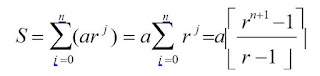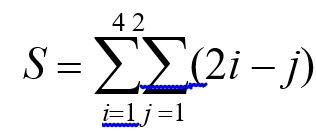## CS6105 Discrete Mathematics ( Final Exam )

The course covers the basic concepts and techniques of discrete mathematics. The course includes the discussion of mathematical logic, propositions, quantifiers, predicates, proof techniques, mathematical induction, fundamentals of set theory, sets, power sets, algebra of sets, relations, functions, count ability and finiteness, graphs and trees.

### Question 1

Marked out of 1.00
Flag question

#### Question text

Let A, B and C represent people who like apples, bananas, and carrots respectively. The number of people in A = 10, B = 12 and C = 16. Three people are such that they enjoy apples, bananas as well as carrots. Two of them like apples and bananas. Let three people like apples and carrots. Also, four people are such that they like bananas and carrots.

How many people like apples only?

How many people like only one of the three?

### Question 2

Marked out of 1.00
Flag question

#### Question text

Find the cardinality of R = {20,21,...,39, 40}

| R | =

### Question 3

Marked out of 1.00
Flag question

#### Question text

A sequence  that involves a common difference in identifying the succeeding terms.

Select one:

### Question 4

Marked out of 1.00
Flag question

#### Question text

A tree is the same as a forest.

Select one:

### Question 5

Marked out of 1.00
Flag question

#### Question text

For a function f : N → N, a recursive definition consists of an initial condition   together with a recurrence relation .

### Question 6

Marked out of 1.00
Flag question

#### Question text

As soon as one vertex of a tree is designated as the root , then every other vertex on the tree can be characterized by its position relative to the root.

### Question 7

Marked out of 1.00
Flag question

#### Question text

Two graphs that are the same are said to be _______________

Select one:

### Question 8

Marked out of 1.00
Flag question

#### Question text

Determine whether the sentence below is an atomic statement, a molecular statement, or not a statement at all.

• The customers wore shoes.

### Question 9

Marked out of 1.00
Flag question

#### Question text

¬P ∨ Q is equivalent to :

Select one:

### Question 10

Marked out of 1.00
Flag question

#### Question text

Two edges are adjacent if they share a vertex.

Select one:

### Question 11

Marked out of 1.00
Flag question

#### Question text

Paths start and stop at the same vertex.

Select one:

### Question 12

Marked out of 1.00
Flag question

#### Question text

IN combinations, the arrangement of the elements is in a specific order.

Select one:

### Question 13

Marked out of 1.00
Flag question

#### Question text

Fill in the blanks.

A graph F is a  if and only if between any pair of vertices in F there is at most  .

### Question 14

Marked out of 1.00
Flag question

#### Question text

Classify the sentence below as an atomic statement, a molecular statement, or not a statement at all. If the statement is molecular, identify what kind it is (conjuction, disjunction, conditional, biconditional, negation).

We can have donuts for dinner, but only if it rains.

### Question 15

Marked out of 1.00
Flag question

#### Question text

A set of statements, one of which is called the conclusion and the rest of which are called premises.

### Question 16

Marked out of 1.00
Flag question

Select one:

### Question 17

Marked out of 1.00
Flag question

#### Question text

Euler paths must touch all edges.

Select one:

### Question 18

Marked out of 1.00
Flag question

#### Question text

An argument is said to be valid if the conclusion must be true whenever the premises are all true.

Select one:

### Question 19

Marked out of 1.00
Flag question

#### Question text

Assume the sequence: 1,3,5,7,9, ….

What is the 20th term?

What type of progression this suggest?

### Question 20

Marked out of 1.00
Flag question

#### Question text

graph has two distinct groups where no vertices in either group connecting to members of their own group

### Question 21

Marked out of 1.00
Flag question

#### Question text

is the simplest style of proof.

### Question 22

Marked out of 1.00
Flag question

#### Question text

In my safe is a sheet of paper with two shapes drawn on it in colored crayon. One is a square, and the other is a triangle. Each shape is drawn in a single color. Suppose you believe me when I tell you that if the square is blue, then the triangle is green. What do you therefore know about the truth value of the following statement?

If the triangle is green, then the square is blue.

### Question 23

Marked out of 1.00
Flag question

#### Question text

Solve for the value of n in :

### Question 24

Marked out of 1.00
Flag question

#### Question text

A   connected graph with no cycles. (If we remove the requirement that the graph is connected, the graph is called a forest.) The vertices in a tree with degree 1 are called  .

### Question 25

Marked out of 1.00
Flag question

#### Question text

Let ‘G’ be a connected planar graph with 20 vertices and the degree of each vertex is 3. Find the number of regions in the graph.

### Question 26

Marked out of 1.00
Flag question

#### Question text

match the following formulas to its corresponding sequenceAnswer 1Choose...Geometric SeriesDouble SummationArithmetic SeriesAnswer 2Choose...Geometric SeriesDouble SummationArithmetic Series

### Question 27

Marked out of 1.00
Flag question

#### Question text

Let A = {1, 2, 3, 4, 5} and B = {3, 4, 5, 6, 7}

Find A ∩ B

Select one:

### Question 28

Marked out of 1.00
Flag question

#### Question text

What is the missing term?

3,9,__,81....

### Question 29

Marked out of 1.00
Flag question

#### Question text

surjective and injecive are opposites  of each other.

Select one:

### Question 30

Marked out of 1.00
Flag question

#### Question text

These are lines or curves that connect vertices.

### Question 31

Marked out of 1.00
Flag question

#### Question text

Find |A ∩ B| when A = {1, 3, 5, 7, 9} and B {2, 4, 6, 8, 10}

### Question 32

Marked out of 1.00
Flag question

#### Question text

Arithmetic progression is the sum of the terms of the arithmetic series.

Select one:

### Question 33

Marked out of 1.00
Flag question

#### Question text

The ________________________ states that if event A can occur in m ways, and event B can occur in n disjoint ways, then the event “A or B” can occur in m + n ways.

Select one:

### Question 34

Marked out of 1.00
Flag question

#### Question text

In my safe is a sheet of paper with two shapes drawn on it in colored crayon. One is a square, and the other is a triangle. Each shape is drawn in a single color. Suppose you believe me when I tell you that if the square is blue, then the triangle is green. What do you therefore know about the truth value of the following statement?

The square and the triangle are both green.

### Question 35

Marked out of 1.00
Flag question

#### Question text

Consider the statement, “If you will give me a cow, then I will give you magic beans.” Determine whether the statement below is the converse, the contrapositive, or neither.

• If I will give you magic beans, then you will give me a cow.

### Question 36

Marked out of 1.00
Flag question

#### Question text

graph has no isolated vertices.

### Question 37

Marked out of 1.00
Flag question

#### Question text

A sequence of vertices such that consecutive vertices (in the sequence) are adjacent (in the graph). A walk in which no edge is repeated is called a trail, and a trail in which no vertex is repeated (except possibly the first and last) is called a path

Select one:

### Question 38

Marked out of 1.00
Flag question

#### Question text

If two vertices are adjacent, then we say one of them is the parent of the other, which is called the  of the parent.

### Question 39

Marked out of 1.00
Flag question

#### Question text

¬(P ∨ Q) is logically equal to which of the following expressions?

Select one:

### Question 40

Marked out of 1.00
Flag question

#### Question text

Suppose P and Q are the statements:

P: Jack passed math.

Q: Jill passed math.

Translate "¬(P ν Q) → Q" into English.

Select one:

### Question 41

Marked out of 1.00
Flag question

Select one:

### Question 42

Marked out of 1.00
Flag question

#### Question text

Let A = {1, 2, 3, 4, 5} and B = {3, 4, 5, 6, 7}

Find A \ B

Select one:

### Question 43

Marked out of 1.00
Flag question

### Question 44

Marked out of 1.00
Flag question

#### Question text

How many 3-letter words with or without meaning, can be formed out of the letters of the word, 'LOGARITHMS', if repetition of letters is not allowed?

### Question 45

Marked out of 1.00
Flag question

#### Question text

Match the following properties of trees to its definition.
 Proposition 4.2.1 Answer 1Choose...Any tree with at least two vertices has at least two vertices of degree one.4 Let T be a tree with v vertices and e edges. Then e  v − 1.A graph F is a forest if and only if between any pair of vertices in F there is at most one pathA graph T is a tree if and only if between every pair of distinct vertices of T there is a unique path. Corollary 4.2.2 Answer 2Choose...Any tree with at least two vertices has at least two vertices of degree one.4 Let T be a tree with v vertices and e edges. Then e  v − 1.A graph F is a forest if and only if between any pair of vertices in F there is at most one pathA graph T is a tree if and only if between every pair of distinct vertices of T there is a unique path. Proposition 4.2.3 Answer 3Choose...Any tree with at least two vertices has at least two vertices of degree one.4 Let T be a tree with v vertices and e edges. Then e  v − 1.A graph F is a forest if and only if between any pair of vertices in F there is at most one pathA graph T is a tree if and only if between every pair of distinct vertices of T there is a unique path. Proposition 4.2.4 Answer 4Choose...Any tree with at least two vertices has at least two vertices of degree one.4 Let T be a tree with v vertices and e edges. Then e  v − 1.A graph F is a forest if and only if between any pair of vertices in F there is at most one pathA graph T is a tree if and only if between every pair of distinct vertices of T there is a unique path.

### Question 46

Marked out of 1.00
Flag question

### Question 47

Marked out of 1.00
Flag question

#### Question text

Let A = {1, 2, 3, 4, 5} and B = {3, 4, 5, 6, 7}

Find A U B

Select one:

### Question 48

Marked out of 1.00
Flag question

Select one:

### Question 49

Marked out of 1.00
Flag question

#### Question text

Tracing all edges on a figure without picking up your pencil or repeating and starting and stopping at different spots

Select one: